Measures of Center MathBitsNotebook.com Terms of Use   Contact Person: Donna Roberts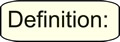A measure of central tendency (measure of center) is a value that attempts to describe a set of data by identifying the central position of the data set (as representative of a "typical" value in the set).

We are familiar with measures of central tendency called the mean, median and mode.

There is another less popular measure of center called the midrange.
The midrange is the average of the maximum and minimum values of the data set,
which is actually the midpoint of the range.
The midrange is not widely used since it is dependent upon only two values in the set.

 Consider: The mean of a distribution can also be thought of as a "balance point" for the distribution. The sum of the distances to the right of the mean equals the sum of the distances to the left of the mean. Think of the mean as the fulcrum for a seesaw. The balancing of the seesaw depends upon the number of items and their distances from the fulcrum.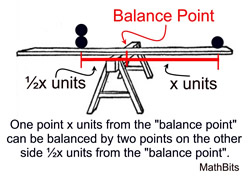Example: Given the data set: {1, 4, 5, 5, 7, 8, 11, 11, 11}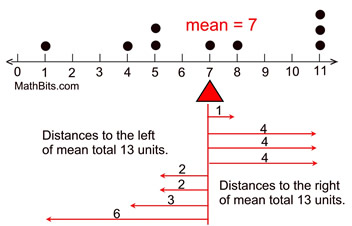Graphically speaking, the center of a distribution is located at the median of the distribution. The median is the point where half of the data points are found on its left side and half on its right side. While the median indicates the "center", it may not always represent the most typical value in the data set. Let's see which measures of center represent the most typical values of the data given various situations.

 Which measures of center are representative of the most typical values in the data set? Distribution Central Tendency Typical Graph Bell-shaped Symmetric Distribution The mean, mode and median will be the same value. • Best measure of center: mean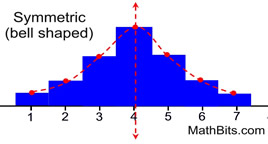Distribution Skewed Right Typically has mean > median There may be exceptions to this statement. • Best measure of center: median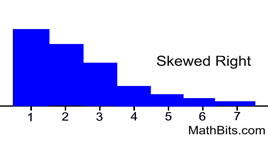Distribution Skewed Left Typically has mean < median There may be exceptions to this statement. • Best measure of center: median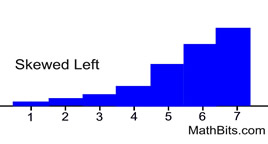U-shaped Symmetric Distribution Neither the mean nor the median is a good indicator of typical values in the set. • Best measure of center: midrange - but the mean as a "balance point" is also a descriptor of the center of the distribution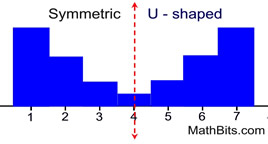Unfortunately, measures of central tendency alone may be insufficient to truly describe the typical data in a set. It is possible that two data sets can have the same mean, but be very different kinds of sets. It is best to use measures of central tendency, along with other observations of the data set, to best describe the data set.